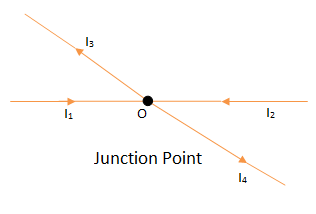# Kirchoff's Voltage Law and Kirchoff's Current Law

### Kirchoff’s Current Law (KCL):Consider a junction point the figure shows the complex network. At this junction point if I1=2A, I2=4A and I3=1A then to find the current I4 we say, sum of current inflowing is 4+4=8A where as sum of current flowing away from the circuit is 2+I4 A.

Thus I4 = 6A.

This study of currents flowing in and out in the circuit is nothing but the use of Kirchhoff’s Current Law. This KCL (kirchoff’s law) can be stated as,

The sum of current flowing towards a junction is equal to the sum of current flowing away from the junction.

This law can also be stated as,

The algebraic sum of all the currents meeting at a point O is always zero. The letter algebraic means in view of the signs of various currents.

ΣI at junction point O = 0

From the above figure the currents I1 and I2are positive while I3 and I4 are negative.
Applying KCL, ΣI at junction point O = 0

I1 +I2-I3-I4 = 0

Ie. I1 + I2 = I3 + I4

This kirchoff’s law is very useful in network simplification.PN Junction Diode

### Kirchoff’s Voltage Law [KVL]:

In any of the network, the algebraic sum of voltage drops across the circuit elements of any closed circuit is equal to the algebraic sum of all the branch voltages, around any closed circuit or closed loop is always zero.

Around a closed path Σv=0

The law states that if one starts at a definite point of a closed path and goes on tracing and noting all the potential changes (either drops or rises), in any one particular direction, till the starting point is reached again, he must be at the same potential with which he started tracing a closed path.

Sum of all the potential rises must be equal to sum of all the potential drops while tracing any closed path of the circuit. The total change in potential along a closed path is always zero.

This law is very useful in loop analysis of the network.Types of Semi Conductor

#### Sign Conventions to be followed while Applying KVL

The voltage drop occurs across the resistance whenever a current flows through resistance. The polarity of this voltage drop is always depends on the direction of current. The current always flow from higher to lower potential.In the Fig-(a), current I is flowing from right to left, therefore point B is higher potential than the point A, as shown in fig.

In the Fig-(b), current I is flowing from left to right, thus point A is at higher potential than the point B, which is also shown.

Once all such polarities are marked in the given circuit, we can apply KVL to any closed path in the circuit.

Now while tracing a closed path, if we go from -ve marked terminal to +ve marked terminal, that voltage must be taken as positive. This is called potential rise.

For example, if the branch AB is traced from A to B then the drop across it must be considered as rise and must be taken as  +IR while writing the equations.

While tracing a closed path, if we go from +ve marked terminal to -ve marked terminal, that voltage must be taken as negative. This is called potential drop.

For example, in the Fig-(a) only, if the branch is traced from B to A then it should be taken as negative, as -IR while writing the equations.

Similarly in the Fig-(b), if branch is traced from A to B then there is a voltage drop and term must be written negative as -IR while writing the equation. U the branch is traced from B to A, it becomes a rise in voltage and term must be written positive as +IR while writing the equation.

In the Fig-(b), current I is flowing from left to right, hence point A is at higher potential than point B, as shown.This post first appeared on My Tech Info, please read the originial post: here

# Share the post

Kirchoff's Voltage Law and Kirchoff's Current Law

×